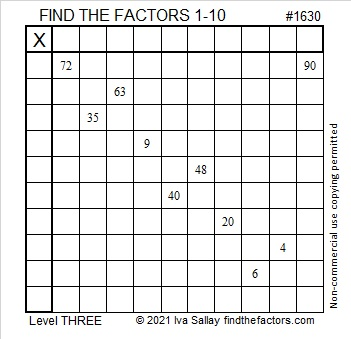# 1630 and Level 3

### Today’s Puzzle:

Write the numbers from 1 to 10 in both the first column and the top row so those numbers and the given clues make the puzzle function like a multiplication table. Because this is a level 3 puzzle, first write the factors for 72 and 90. Then work your way down the puzzle row by row until you have found all the factors.### Factors of 1630:

• 1630 is a composite number.
• Prime factorization: 1630 = 2 × 5 × 163.
• 1630 has no exponents greater than 1 in its prime factorization, so √1630 cannot be simplified.
• The exponents in the prime factorization are 1, 1, and 1. Adding one to each exponent and multiplying we get (1 + 1)(1 + 1)(1 + 1) = 2 × 2 × 2 = 8. Therefore 1630 has exactly 8 factors.
• The factors of 1630 are outlined with their factor pair partners in the graphic below.### More about the Number 1630:

1630 is the hypotenuse of a Pythagorean triple:
978-1304-1630, which is (3-4-5) times 326.